×#### Thank you for registering.

One of our academic counsellors will contact you within 1 working day.

Click to Chat

1800-1023-196

+91-120-4616500

CART 0

• 0

MY CART (5)

Use Coupon: CART20 and get 20% off on all online Study Material

ITEM
DETAILS
MRP
DISCOUNT
FINAL PRICE
Total Price: Rs.

There are no items in this cart.
Continue Shopping• Complete JEE Main/Advanced Course and Test Series
• OFFERED PRICE: Rs. 15,900
• View Details

```Chapter 17: Constructions Exercise – 17.2

Question: 1

Draw an angle and label it as ∠BAC. Construct another angle, equal to ∠BAC

Solution: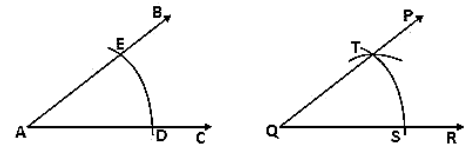Steps of construction:

1. Draw an angle ABC and a line segment QR.

2. With center A and any radius, draw an arc which intersects ∠BAC at E and D.

3. With Q as a centre and same radius draw an arc which intersects QR at S.

4. With S as center and radius equal to DE, draw an arc which intersects the previous arc at T.

5. Draw a line segment joining Q and T.

Therefore ∠PQR = ∠BAC

Question: 2

Draw an obtuse angle. Bisect it. Measure each of the angles so formed.

Solution: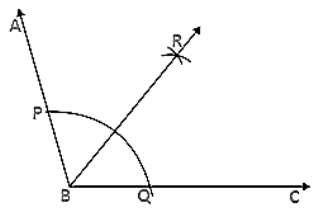Steps of construction:

1. Draw an angle ∠ABC of 120°.

2. With B as a centre and any radius, draw an arc which intersects AB at P and BC at Q.

3. With P as center and radius more than half of PQ draw an arc.

4. With Q as a center and same radius draw an arc which cuts the previous arc at R.

5. Join BR.

Therefore ∠ABR = ∠RBC = 60°

Question: 3

Using your protractor, draw an angle of 108°. With this given angle as given, draw an angle of 54°.

Solution: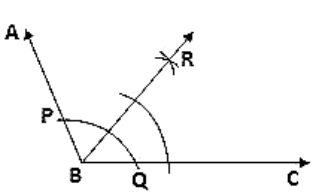Steps of construction:

1. Draw an angle ABC of 108°.

2. With B as the center and any radius draw an arc which intersects AB at P and BC at Q.

3. With P as center and radius more than half of PQ draw an arc.

4. With Q as the centre and same radius draw an arc which intersects the previous arc at R.

5. Join BR.

Therefore ∠RBC = 54°

Question: 4

Using the protractor, draw a right angle. Bisect it to get an angle of measure 45°.

Solution: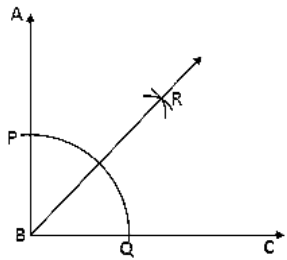Steps of construction:

1. Draw an angle ABC of 90°.

2. With B as the centre and any radius draw an arc which intersects AB at P and BC at Q.

3. With P as center and radius more than half of PQ draw an arc.

4. With Q as center and same radius draw an arc which intersects the previous arc at R.

5. Join RB.

Therefore ∠RBC = 45°

Question: 5

Draw a linear pair of angles. Bisect each of the two angles. Verify that the two bisecting rays are perpendicular to each other.

Solution:Steps of construction:

1. Draw two angles DCA and DCB forming linear pair

2. With center C and any radius draw an arc which intersects AC at P and CD at Q and CB at R

3. With center P and Q and any radius draw two arcs which intersect each other at S

4. Join SC

5. With Q and R as center and any radius draw two arcs which intersect each other at T

6. Join TC

Therefore ∠SCT = 90°.

Question: 6

Draw a pair of vertically opposite angles. Bisect each of the two angles. Verify that the bisecting rays are in the same line.

Solution: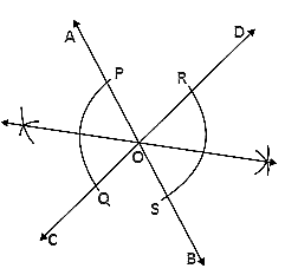Steps of Construction:

1. Draw a pair of vertically opposite angle ∠AOC and ∠DOB.

2. Keeping O as the center and any radius draw two arcs which intersect OA at P, OC at Q, OB at S and OD at R.

3. Keeping P and Q as center and radius more than half of PQ draw two arcs which intersect each other at T.

4. Join TO.

5. Keeping R and S as center and radius more than half of RS draw two arcs which intersect each other at U.

6. Join OU.

Therefore TOU is a straight line

Question: 7

Using rulers and compasses only, draw a right angle.

Solution:Steps of construction:

1. Draw a line segment AB.

2. Keeping A as the center and any radius draw an arc which intersects AB at C.

3. Keeping C as center and the same radius draw an arc which intersects the previous arc at D.

4. Keeping D as the center and same radius draw an arc which intersects arc in (2) at E.

5. Keeping E and D as center and radius more than half of ED draw arcs which intersect each other at F.

6. Join FA.

Therefore ∠FAB = 90°

Question: 8

Using rulers and compasses only, draw an angle of measure 135°.

Solution:Steps of construction:

1. Draw a line segment AB and produce BA to C.

2. Keeping A as the center and any radius draw an arc which intersects AC at D and AB at E.

3. Keeping D and E as center and radius more than half of DE draw arcs which intersect each other at F.

4. Join FA which intersects the arc in (2) at G.

5. Keeping G and D as center and radius more than half of GD draw arcs which intersect each other at H.

6. Join HA.

Therefore ∠HAB = 135°

Question: 9

Using a protractor, draw an angle of measure 72°. With this angle as given draw angles of measure 36° and 54°.

Solution:Steps of construction:

1. Draw an ∠ABC of 720 with the help of a protractor.

2. Keeping B as center and any radius draw an arc which intersects AB at D and BC at E.

3. Keeping D and E as center and radius more than half of DE draw two arcs which intersect each other at F.

4. Join FB which intersects the arc in (2) at G.

5. Keeping D and G as center and radius more than half of DG draw two arcs which intersect each other at H

6. Join HB

Therefore ∠HBC = 54° ∠FBC = 36°

Question: 10

Construct the following angles at the initial point of a given ray and justify the construction:

(i) 45°

(ii) 90°

Solution:

(i) Steps of construction: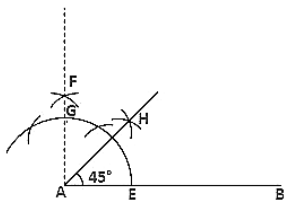1. Draw a line segment AB and produce BA to C.

2. Keeping A as the center and any radius draw an arc which intersects AC at D and AB at E.

3. Keeping D and E as center and radius more than half of DE draw arcs which intersect each other at F.

4. Join FA which intersects the arc in (2) at G.

5. Keeping G and E as center and radius more than half of GE draw arcs which intersect each other at H.

6. Join HA.

Therefore ∠HAB = 45°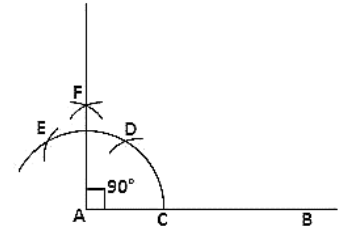(ii) Steps of construction

1. Draw a line segment AB.

2. Keeping A as the center and any radius draw an arc which intersects AB at C.

3. Keeping C as center and the same radius draw an arc which intersects the previous arc at D.

4. Keeping D as the center and same radius draw an arc which intersects arc in (2) at E.

5. Keeping E and D as center and radius more than half of ED draw arcs which intersect each other at F.

6. Join FA.

Therefore ∠FAB = 90°

Question: 11

Construct the angles of the following measurements:

(i) 30°

(ii) 75°

(iii) 105°

(iv) 135°

(v) 15°

(vi) 22(1/2)°

Solution:(i) Steps of construction:

1. Draw a line segment AB.

2. Keeping A as the centre and any radius draw an arc which intersects AB at C.

3. Keeping C as center and the same radius draw an arc which intersects the previous arc at D.

4. Keeping D and C as center and radius more than half of DC draw arcs which intersect each other at E.

5. Join EA.

Therefore ∠EAB = 30°

(ii) Steps of construction:1. Draw a line segment AB

2. Keeping A as center and any radius draw an arc which intersects AB at C

3. Keeping C as center and the same radius draw an arc which intersects the previous arc at D

4. Keeping D as center and same radius draw an arc which intersects arc in (2) at E

5. Keeping E and D as center and radius more than half of ED, draw arcs intersecting each other at F

6. Join FA which intersects arc in (2) at G

7. Keeping G and D as center and radius more than half of GD draw arcs intersecting each other at H

8. Join HA

Therefore ∠HAB = 75°

(iii) Steps of construction:1. Draw a line segment AB.

2. Keeping A as the center and any radius draw an arc which intersects AB at C.

3. Keeping C as center and the same radius draw an arc which intersects the previous arc at D.

4. Keeping D as the centre and same radius draw an arc which intersects arc in (2) at E.

5. Keeping E and D as center and radius more than half of ED draw arcs which intersect each other at F.

6. Join FA which intersects arc in (2) at G.

7. Keeping E and G as center and radius more than half of EG draw arcs which intersect each other at H.

8. Join HA.

Therefore ∠HAB = 105°

(iv)  Steps of construction:1. Draw a line segment AB and produce BA to C.

2. Keeping A as the center and any radius draw an arc which intersects AC at D and AB at E.

3. Keeping D and E as center and radius more than half of DE draw arcs which intersect each other at F.

4. Join FA which intersects the arc in (2) at G.

5. Keeping G and D as center and radius more than half of GD draw arcs which intersect each other at H

6. Join HA.

Therefore ∠HAB = 135°

(v) Steps of construction: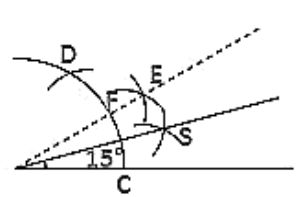1. Draw a line segment AB.

2. Keeping A as the centre and any radius draw an arc which intersects AB at C.

3. Keeping C as center and the same radius draw an arc which intersects the previous arc at D.

4. Keeping D and C as center and radius more than half of DC draw arcs which intersect each other at E.

5. Join EA which intersects arc in (2) at F.

6. Keeping F and C as center and radius more than half of FC draw arcs which intersect each other at G.

7. Join GA.

Therefore ∠GAB = 15°

(vi) Steps of construction: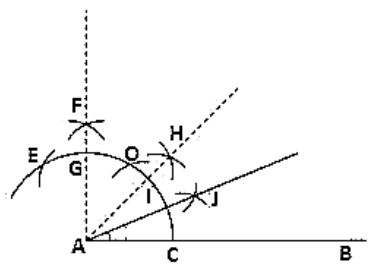1. Draw a line segment AB.

2. Keeping A as the center and any radius draw an arc which intersects AB at C.

3. Keeping C as center and the same radius draw an arc which intersects the previous arc at D.

4. Keeping D as the centre and same radius draw an arc which intersects arc in (2) at E.

5. Keeping E and D as center and radius more than half of ED draw arcs which intersect each at F.

6. Join FA which intersects arc in (2) at G.

7. Keeping G and C as center and radius more than half of GC draw arcs intersecting each other at point H.

8. Join HA which intersects the arc in (2) at a point I.

9. Keeping I and C as center and radius more than half of IC draw arcs intersecting each other at point J.

10. Join JA.

Therefore ∠JAB = 22(1/2)°
```### Course Features

• 728 Video Lectures
• Revision Notes
• Previous Year Papers
• Mind Map
• Study Planner
• NCERT Solutions
• Discussion Forum
• Test paper with Video Solution